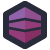The transition from Python 2 to Python 3 has been a long running challenge. Arguably, too long running, but those are the challenges when you introduce breaking changes into a widely used language.

Now, one of those breaking changes was around string handling and encoding- Unicode versus ASCII. This meant that, if you wanted code to run on both versions of Python, you'd need to check which version you were on to handle encodings properly.

Ryan J's predecessor wanted to implement logic where, on Python 2, they just handled the strings without transformation, while in Python 3, they'd force the string to be encoded in "latin1". The traditional way of writing this would be something like:

``````if sys.version_info < 3:
_b = lambda(x: x)
else:
_b = lambda(x: x.encode('latin1')
``````

Now, anyplace you have to handle a string, you can `stringVar = _b(stringVar)`. On Python 2 it's a no-op, on Python 3 it re-encodes the string. It's maybe not the most elegant or Pythonic way of solving the problem, but it's a common enough practice.

But that doesn't show off how clever you are. It needs to be a one-liner. You could do something like this, using Python's version of a ternary:

``````_b = (lambda x: x) if (sys.version_info < 3) else (lambda x: x.encode('latin1'))
``````

But that's not clever enough. No, you have to show your absolute mastery over the language, using constructs to do things they were never intended to do.

``````_b=sys.version_info<3 and (lambda x:x) or (lambda x:x.encode('latin1'))
``````

There we go. This exploits the fact that boolean functions don't return boolean types- they return the non-falsy value that satisfied their condition.

Let's walk through it and start with the `and`. If we're on Python 2, the first term of the `and` is true, so we have to evaluate the second. Now a lambda is always going to be truth-y, so the `and` is satisfied and is true. We don't need to evaluate the `or`, because regardless of what the second expression is, the whole thing is true. So this returns `(lambda x:x)` and stores it in `_b`.

If we're on Python 3, then the entire `and` is false, so we don't need to look at the second term in the `and`. But the `or` now matters, so we evaluate the second term in the `or`, and thus return `(lambda x:x.encode('latin1'))`.

Code where you need to write out a truth-table to understand its operation is way more clever than a basic conditional expression, thus this code succeeds at its core goal: establishing that the author was Very Smart™.[Advertisement] Continuously monitor your servers for configuration changes, and report when there's configuration drift. Get started with Otter today!